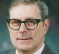## Radar: Polar to cartesian conversion.Started by 2 years ago4 replieslatest reply 2 years ago440 views

Hi.  Something that I have been kicking around for a while is (probably) an Arm based user-side open-source "black box" module to re-use an older ship radar, with a modern colour display.  This would involve a polar to cartesian converter, echo-strength(colour), and zoom function.  The HV and detection side, AFAIK, would be relatively easy.  There is already an open-source chartplotter (openCPN) on the go.  I think there is an ARM processor with built-in trigonometry functions.

Would anyone care to comment ?

TIA

[ - ]Conversion is quite simple -https://en.wikipedia.org/wiki/Polar_coordinate_sys...

#include <iostream>// helpful source: https://www.mathsisfun.com/polar-cartesian-coordin...#include <cmath>using namespace std;int main() {    //float r = 2000.0f;  // distance    //float a = 33.25f;   // angle        bool angleInDegrees = true;        float r = { 13.0f, 12.0f, 2001.45f, 7000.5f }; // indexes (0, 1, 2, 3)    float a = { 22.6f, 195.0f, 335.1342f, 60.3412f };    int n = size(r);        for(int i = 0; i < n; i++){        if(angleInDegrees){            a[i] = a[i] * (float)(M_PI/180.0);        }        float x = r[i] * std::cos(a[i]);        float y = r[i] * std::sin(a[i]);                printf("Angle = %3.5f rad  Distance = %5.3f m  -->  x = %5.3f m  y = %5.3f m\n", a[i], r[i], x, y );    }        return 0;}

You can test drive code at https://www.codechef.com/ide

[ - ]I understand the math, but the rest of it would be very time-consuming for a limp-along coder.  I can just about manage with an Arduino using their IDE, but I suspect that a Pi or Beagle-Bone would be appropriate for this job. One of these years ....
[ - ]Define a rectangular coordinate system with the radar at the origin. By definition, the center of the beam is a zero-thickness ray. Let all the coordinate points be positive integers. Choose a maximum integer L. Initially the ray points from (0,0) to (0,1). At the same time it also points to (0,2) and to (0,3) and ... and to (0,L). These are each at angle 0 in the coordinate system.

My algorithm generates all the coordinate pairs in a triangle, in their natural polar coordinate order, ending at (1,1) - which is at the same angle as (2,2), ... , as (L,L). The algorithm is super fast and uses utterly negligible memory.

Then, as you get the data from the radar, you can either copy the radar data into each cartesian coordinate pair from the nearest polar pair, or interpolate from the nearest two polar coordinate measurements.

This, of course, gets you only cartesian coordinates in a 45 degree triangle, but the same algorithm can then be run backwards and the resulting coordinate pairs can be reflected to get all the coordinate pairs in angular order from (1,1) to (0,1). Similar tricks can fill in the other 6 triangular regions with the same basic algorithm and reflections and sign changes.

If the radar angular scan begins and or ends at some angle other than 0, there's no extra cost for starting or ending at any arbitrary angle.

My basic algorithm requires only a few integer operations per point. It's documented in, among other places, a minichapter I contributed to Rick Lyons book, "Streamlining Digital Signal Processing, IEEE Press, 2007, p 277-283

I'm not aware of anybody except me ever using it.

If memory is no limitation, one could just pre-build a table of Cartesian coordinates in their natural angular order (which is essentially what my algorithm ends up doing).

[ - ]